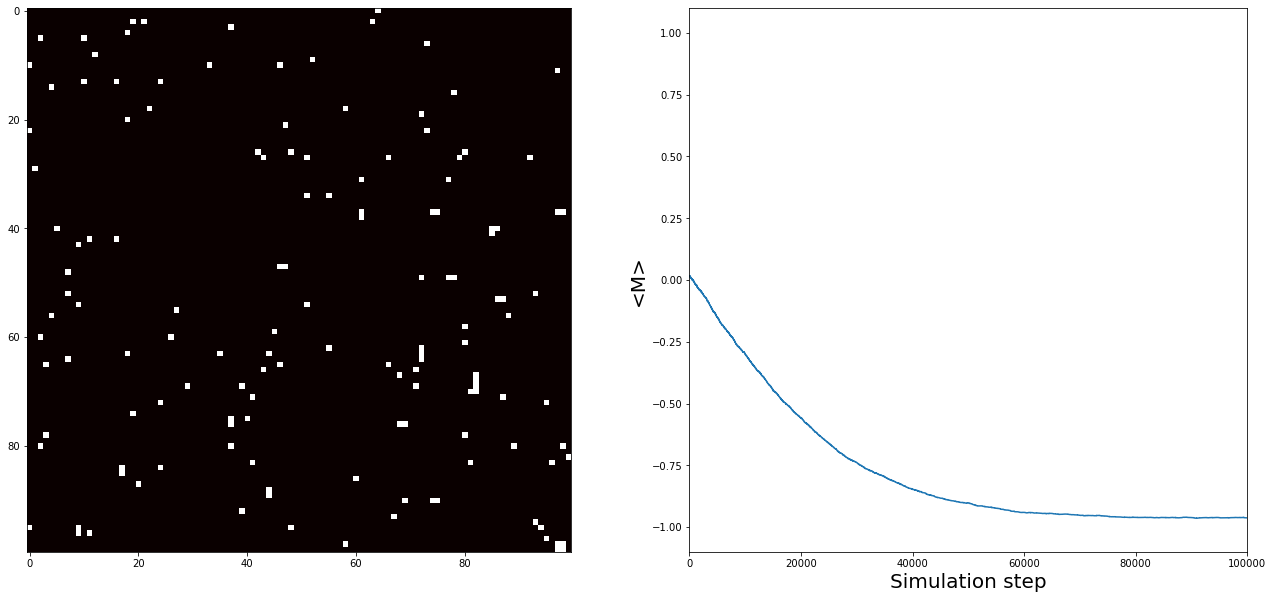# The Ising model¶

## Goal:¶

Implementing computational simulation of one dimension Ising model

### history¶

This model was suggested to Ising by his thesis adviser, Lenz. Ising solved the one-dimensional model, ..., and on the basis of the fact that the one-dimensional model had no phase transition, he asserted that there was no phase transition in any dimension. As we shall see, this is false. It is ironic that on the basis of an elementary calculation and erroneous conclusion, Ising’s name has become among the most commonly mentioned in the theoretical physics literature. But history has had its revenge. Ising’s name, which is correctly pronounced “E-zing,” is almost universally mispronounced “I-zing.”

Barry Simon

#### For revising the theoritical concept of ising model including derivation and all other aspects, I recommend going through "Statistical Mechanics 3rd Edition" section 13.2¶

In :
import numpy
import matplotlib.pyplot as plt

## The following package is required to update the plot within the same figure
## this allows us to save time editing plot without the need to re-run code
from IPython.display import clear_output


To update our two plots during the simulation we have in this notebook we use the function live_plot in the following cell. it allows us to do this through updating the plot in a sequence.

In :
def live_plot(data_2D, data_1D):
"""
Clear the figure and update the plots with newly created data

:params:  - data_2D --> 2D numpy array
- data_1D --> Two 1D numpy arrays containing the x and y axis of the plotted data
"""
clear_output(wait=True)

## we initialize the figure with corresponding subplots
fig = plt.figure(figsize = (22, 10))

## we plot direct visualization of the spin states in the system
ax1.imshow(data_2D, interpolation = "none", vmin = -1., vmax = 1., cmap = "hot")

## we plot magnetization as a function of time. where time here corresponds to simulation steps
ax2.plot(data_1D[:, 0], data_1D[:, 1])
ax2.set_xlim(0, Steps)
ax2.set_ylim(-1.1, 1.1)

ax2.set_xlabel("Simulation step", fontsize = 20)
ax2.set_ylabel("<M>", fontsize = 20)

plt.show();


Let start with defining system parameters by defining the number of particles in our system N_ and the number of Steps_ the simulation is executed over.

The thermal energy k_BT and the external magnetic field B_ are expressed in terms of the nearest neighbour exchange energy J.

In :
N_ = 100
Steps_ = 300000

B_ = -1.0          # [J]
k_BT = 2.3        # [J]

## Calculate the energy change due to one spin flip
def deltaE(S, i, j):
Sij  = S[i, j]
Enow = -Sij * (S[(i+1)%N_, j] + S[(i-1)%N_, j] + S[i, (j+1)%N_] + S[i, (j-1)%N_] + float(B_)) ## Use periodic boundary conditions
return -2 * Enow


Now we initialize the system by randomly defining the spins. We do this by generating a two by two array with values between 0 and 1. All values below 0.5 correspond to spin down, -1 and all values above 0.5 correspond to spin up, +1.

In :
## Initially, we assign the spins randomly
spins = numpy.where(numpy.random.random((N_, N_)) > 0.5, 1, -1)

## Determine the magnitization and store in numpy array with shape:
M = numpy.zeros((Steps_, 2))
M = 0, numpy.array([numpy.mean(spins)])

## Now we define what every simulation step will do
for idx in range(Steps_):
## Select a random value for i and j
i, j = numpy.random.randint(0, N_, 2)
## For the selected indices i and j determine the change in energy
dE = deltaE(spins, i, j)

## If the change in energy is smaller than 0, or a random number is smaller
## than the corresponding thermal fluctuations, allow the spin change/flip
if (dE < 0.0) or (numpy.random.random() < numpy.exp(-dE / float(k_BT))):
spins[i, j] = -spins[i, j]

## Determine the magnetization of the system and store into the
M[idx] = idx, numpy.mean(spins)

## For every 999th step, update the plots
if idx %(999) == 0:
live_plot(spins, M[:idx])In [ ]: